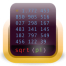# Maple

by Waterloo Maple

## A mathematics software used to analyze and quickly solve the problems

Operating system: Windows

Publisher: Waterloo Maple

Release: Maple 2018.2.0.0

Antivirus check: passed

Last revision: Last week

Maple is different and useful software for the persons who are in math and engineering field. It helps to quickly solve the math related problems quickly and efficiently. It is designed in such a way that it gives a solution to maximum math problems in quite a few steps.

Features:

• Solve different math functions like Differential equations, Tolerances etc.
• The coding is very easy
• It uses simple and reliable interface
• The work can be encrypted so that it will not be deleted easily.
• Able to solve more partial differential equations with special conditions.
• Easy to integrate units in the software so that it can be used in future.
• Can be used in different fields including Physics, Financial Modeling, Signal Processing.
• Content protection is available to save the date in a secure way.

Maple is a cutting-edge software used in the calculation of math-related problems quickly and easily. It is mostly used by engineers in the field of construction, aeronautical. Nowadays this software is also used by students to practice math problems and solve them quickly so that it would be easy during their tests.

Helps to solve math problems quickly.

Maple has many features related to math as it is specially designed to solve math problems. It is time consuming when solving critical math problems like partial differential equations with special conditions, differential equations, physics, financial modeling, signal processing. More complex units are saved in the software so that it can be used for a future solution for problems.

There is a package known as Group Theory package which consists of equations and formula which can be frequently used in solving problems. The Graph Theory has essential elements which are used in drawing and computing of graphical problems. The interface is very simple for the user to use the software.

John
This is a software that a lot of users use to learn math on. It acts as somewhat of a tutor. IT makes it easier to learn new mathematical calculations. This can be downloaded on Windows and Linux, but it is less popular on Mac book. You can download service packs that have constant new updates and new ways to bring on better service.SA data analysis and visualization softwareA software used for numerical computing and graphingFor all quick math wizzesA great simple math program that can help you solve challenging math problemsCreate and insert mathematical equations into word documents